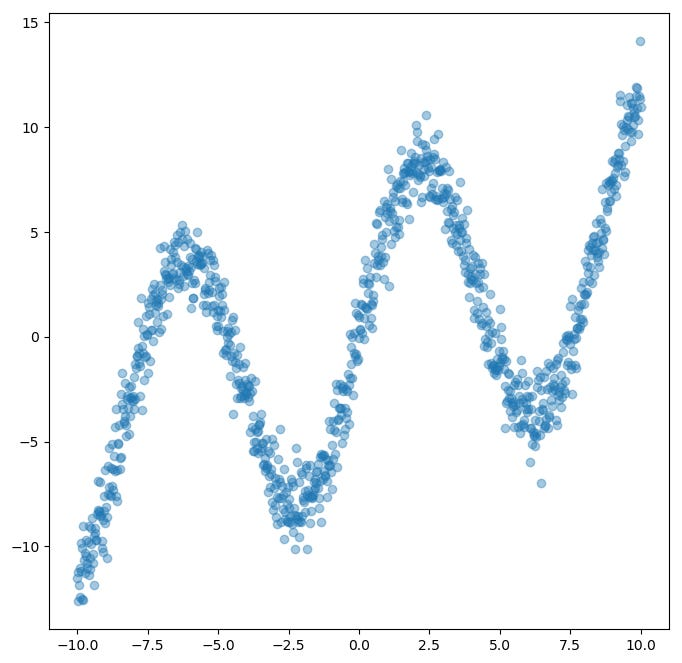# Deep Learning Cage Match: Multi-layer NN vs Mixture Density network

Unless you have been living on a desert island the past few years, you have probably heard of neural networks. An old technique, made new again through the magic of modern hardware and innovations like batch normalization and he-initialization that seems to be.. in a word.. unstoppable.

However, mathematicians also developed a plethora of other tools and techniques also over the years, one of the most powerful being variational inference.

The key idea behind variational inference is that any data that has a pattern, can be thought of as being drawn from a probability distribution, lets call it P. variational inference tries to infer or learn an approximation of P, called Q.

A common approach to learning Q, is to use a “mixture model”. A mixture model can be thought of as a bunch of gaussians, with different means, and variances. To reproduce the pattern in the data, we sample from Q. If Q is a close approximation of P, then the data sampled from Q will be indistinguishable from P.

The job now becomes, figure out a good set of parameters, ie: those means and variances, for Q.

Well, I know something that is good at figuring out what the values of parameters should be… a neural net! This is the idea behind the Mixture Density Network.

Ok, you say.. sounds cool and all but… why? Isn’t this all a bit indirect? Why would we use a NN to estimate a probability distribution that approximates our ground truth, when we could just infer it directly with a NN?

Well, for Science! Let’s try out both methods and see what happens.

Let’s picky a tricky distribution, the humble sine wave.

Now, lets train a neural network to approximate this distribution.

As you can see, the network falls directly into an average state, and cannot break out. This is due to the mean squared error loss function, which has a large “ground state” around the average of the sine wave. It’s not impossible for the network to fit this function, but it’s not easy.

Now lets see how the mixture density model deals with this.

Well, that’s different! Here you can see the model starts by increasing variance to cover the whole function, then the variance tightens up towards the end, until the approximation fits the data well.

Well, for this toy problem. Mixture Density Wins!

You can check out the code of MDN on github!

Source: Deep Learning on Medium